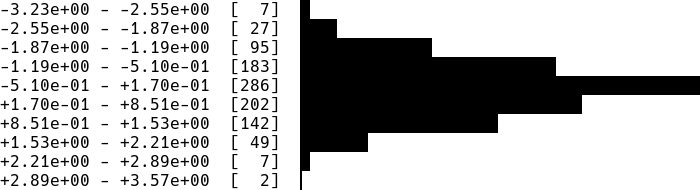Showing:

0

374

3mos ago

7

0

# termplotlib

termplotlib is a Python library for all your terminal plotting needs. It aims to work like matplotlib.

### Line plots

For line plots, termplotlib relies on gnuplot. With that installed, the code

``````import termplotlib as tpl
import numpy

x = numpy.linspace(0, 2 * numpy.pi, 10)
y = numpy.sin(x)

fig = tpl.figure()
fig.plot(x, y, label="data", width=50, height=15)
fig.show()
``````

produces

``````    1 +---------------------------------------+
0.8 |    **     **                          |
0.6 |   *         **           data ******* |
0.4 | **                                    |
0.2 |*              **                      |
0 |                 **                    |
|                                   *   |
-0.2 |                   **            **    |
-0.4 |                     **         *      |
-0.6 |                              **       |
-0.8 |                       **** **         |
-1 +---------------------------------------+
0     1    2     3     4     5    6     7
``````

### Horizontal histograms

``````import termplotlib as tpl
import numpy

numpy.random.seed(123)
sample = numpy.random.normal(size=1000)
counts, bin_edges = numpy.histogram(sample)

fig = tpl.figure()
fig.hist(counts, bin_edges, orientation="horizontal", force_ascii=False)
fig.show()
``````

producesHorizontal bar charts are covered as well. This

``````import termplotlib as tpl

fig = tpl.figure()
fig.barh([3, 10, 5, 2], ["Cats", "Dogs", "Cows", "Geese"], force_ascii=True)
fig.show()
``````

produces

``````Cats   [ 3]  ************
Dogs     ****************************************
Cows   [ 5]  ********************
Geese  [ 2]  ********
``````

### Vertical histograms

``````import termplotlib as tpl
import numpy

numpy.random.seed(123)
sample = numpy.random.normal(size=1000)
counts, bin_edges = numpy.histogram(sample, bins=40)
fig = tpl.figure()
fig.hist(counts, bin_edges, grid=[15, 25], force_ascii=False)
fig.show()
``````

produces### Tables

Support for tables has moved over to termtables.

### Installation

termplotlib is available from the Python Package Index, so simply do

``````pip install termplotlib
``````

to install.

### Testing

To run the termplotlib unit tests, check out this repository and type

``````pytest
``````

### Similar projects

This software is published under the GPLv3 license.

## Rate & Review

Great Documentation0
Easy to Use0
Performant0
Highly Customizable0
Bleeding Edge0
Responsive Maintainers0
Poor Documentation0
Hard to Use0
Slow0
Buggy0
Abandoned0
Unwelcoming Community0
100